Download Presentation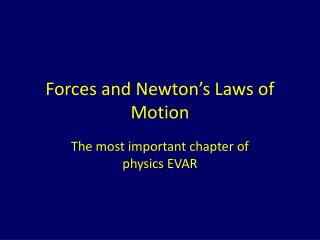Forces and Newton’s Laws of Motion

# Forces and Newton’s Laws of Motion - PowerPoint PPT Presentation

Forces and Newton’s Laws of Motion. The most important chapter of physics EVAR. What is a Force?. A force is a push or a pull Contact forces: Boat pulling a water skier Pushing a car with a dead battery Noncontact forces: Gravitational attraction of moon to earthI am the owner, or an agent authorized to act on behalf of the owner, of the copyrighted work described.
Download Presentation## Forces and Newton’s Laws of Motion

An Image/Link below is provided (as is) to download presentation

Download Policy: Content on the Website is provided to you AS IS for your information and personal use and may not be sold / licensed / shared on other websites without getting consent from its author.While downloading, if for some reason you are not able to download a presentation, the publisher may have deleted the file from their server.

- - - - - - - - - - - - - - - - - - - - - - - - - - E N D - - - - - - - - - - - - - - - - - - - - - - - - - -
Presentation Transcript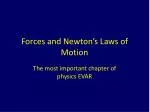### Forces and Newton’s Laws of Motion

The most important chapter of physics EVAR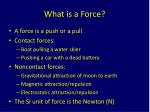What is a Force?
• A force is a push or a pull
• Contact forces:
• Boat pulling a water skier
• Pushing a car with a dead battery
• Noncontact forces:
• Gravitational attraction of moon to earth
• Magnetic attraction/repulsion
• Electrostatic attraction/repulsion
• The SI unit of force is the Newton (N)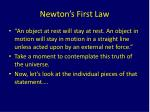Newton’s First Law
• “An object at rest will stay at rest. An object in motion will stay in motion in a straight line unless acted upon by an external net force.”
• Take a moment to contemplate this truth of the universe.
• Now, let’s look at the individual pieces of that statement….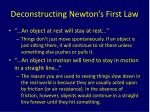Deconstructing Newton’s First Law
• “…An object at rest will stay at rest…”
• Things don’t just move spontaneously. If an object is just sitting there, it will continue to sit there unless something else pushes or pulls it.
• “…An object in motion will tend to stay in motion in a straight line…”
• The reason you are used to seeing things slow down in the real world is because they are usually acted upon by friction (or air resistance). In the absence of friction, however, objects would continue in a straight line forever until they hit something.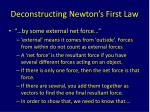Deconstructing Newton’s First Law
• “…by some external net force…”
• ‘external’ means it comes from ‘outside’. Forces from within do not count as external forces
• A ‘net force’ is the resultant force if you have several different forces acting on an object.
• If there is only one force, then the net force is that force.
• If there are several, you add them together as vectors to find the one final resultant force.
• In order to show that, we need to know that….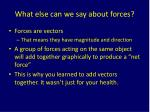What else can we say about forces?
• Forces are vectors
• That means they have magnitude and direction
• A group of forces acting on the same object will add together graphically to produce a “net force”
• This is why you learned to add vectors together. It wasn’t just for your health.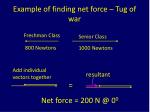Example of finding net force – Tug of war

Freshman Class

Senior Class

800 Newtons

1000 Newtons

vectors together

resultant

=

Net force = 200 N @ 00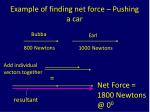Example of finding net force – Pushing a car

Bubba

Earl

800 Newtons

1000 Newtons

vectors together

=

Net Force =

1800 Newtons

@ 00

resultant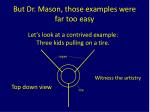But Dr. Mason, those examples were far too easy

Let’s look at a contrived example:

Three kids pulling on a tire.

ropes

Witness the artistry

Top down view

tire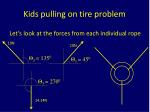Kids pulling on tire problem

Let’s look at the forces from each individual rope

10N

10N

Θ2 = 1350

Θ1 = 450

Θ3 = 2700

14.14NKids pulling on tire problem

What are the horizontal and vertical components

of each force?

10N

F = 10N

FY = 7.07N

Θ1 = 450

FX = 7.07N

Find the horizontal and vertical components of

force the same way you do for other vectors.Kids pulling on tire problem

What are the horizontal and vertical components

of each force?

F = 10N

10N

FY = 7.07N

Θ2 = 1350

FX = -7.07NNow let’s look at all the forces again
• Forces acting horizontally:
• X-component of force 1 = 7.07N
• X-component of force 2 = -7.07N
• X-component of force 3 = 0 N
• Forces acting vertically:
• Y-component of force 1 = 7.07N
• Y-component of force 2 = 7.07N
• Y-component of force 3 = -14.14 N
• So, all forces in the X-direction add to Zero and all forces in Y-direction add to Zero
• So net force on tire is Zero NewtonsInertia and Mass
• Inertia: the tendency for an object to remain at rest or in motion in a straight line.
• Mass: a quantitative measure of inertia
• SI unit for inertia/mass: kilogram (kg)
• We will discuss mass at length shortlyNewton’s Second Law
• This is the most important physics law you will learn.
• It is way more important than that E=MC2 business.
• You ready for it?
• Here it is:
• F = MA
• Force = mass X accelerationInvestigating Newton’s Second Law
• F = ma
• Or, more specifically, ΣF = ma
• Where ‘Σ’ = the greek letter “sigma” and means “sum of”
• So the sum of all the forces on an object are equal to that objects mass times its acceleration
• As before, the forces on an object add together vectorally. This is how you find the resultant.Examples of Newton’s 2nd law
• You are pushing a 200 kg sled over a completely frictionless frozen lake. Your applied force is 150 N. What is the sled’s acceleration?
• F = ma -> 150 N = (200 kg)(a)
• a = (150 N)/(200 kg) = 0.75 m/s2More Examples of Newton’s 2nd law
• Now, you are pushing a 200 kg sled over a frozen lake with some friction. Your applied force is still 150 N, but now it is counteracted by a 100 N friction force. What is the sled’s acceleration?
• ΣF = ma
• So, 150 N – 100 N = ma
• 50 N = (200 kg)(a)
• a = (50 N)/(200 kg) = 0.25 m/s2Mini-competition 1: Question 1
• Chuck Norris (who once successfully ordered a Big Mac at Burger King) has a fist of mass 0.7kg. Starting from rest, his fist achieves a velocity of 8m/s in 0.15 sec. What avg force does he generate to achieve this level of performance?
• 37 N
• If paper beats rock, rock beats scissors, and scissors beats paper, what beats all 3 at the same time?
• Chuck Norris.Mini-competition 1: Question 2
• Carla Wong serves a 58 gram tennis ball and accelerates it from rest to 45m/s. The impact gives it a constant acceleration over 44cm. What is the magnitude of the force on the ball?
• 133 NNewton’s 3rd Law
• “Whenever one body exerts a force on a second body, the second body exerts an oppositely directed force of equal magnitude on the first body.”
• Whenever you push on something, that something pushes back on you with the same force.Newton’s Third law example
• From the book:
• An astronaut (92kg) pushes on a satellite (11,000kg) with a force of 36N. What is the accel of the astronaut? Of the Satellite?
• Fastronaut on satellite = - Fsatellite on astronaut
• Aastronaut = -F/mastronaut = -36N/92kg = -0.30m/s2
• Asatellite = F/msatellite = 36N/11,000kg = 0.0033m/s2Mini-competition 2: Question 1
• Charles Steigerwald pushes on a wall to the right with a force of 200 N. What is the force that the wall exerts on Charles?
• -200NMini-competition 2: Question 2
• Kurt Stegmeier (mass 63 kg) and Dr. Mason (mass 110 Kg) are sitting opposite one another in rolling chairs. They push off one another in opposite directions with a force of 100 N. Assuming perfectly frictionless school chairs and floors, what is the magnitude of the acceleration each experiences?
• Dr. Mason: 0.909 m/s2
• Kurt: 1.58 m/s2Gravitational Force
• Gravitational force is always attractive
• Fg = Gm1m2/r2
• Fg = force of gravity
• M1 = mass of first object
• M2 = mass of second object
• r = distance between them (or specifically, between their centers of mass
• G = 6.67X10-11 Nm2/kg2A note on the center of mass
• Every object has a center of mass
• For purposes of calculating forces like gravity, you can assume that all the mass of the object is at the center of mass.
• For a sphere (assuming uniform density), center of mass is the actual center

r

is

like

r

m1

m2

m1

m2Gravitational Force example 1
• What is magnitude of gravitational force between two particles of masses m1 = 12kg and m2 = 25 kg separated by distance r = 1.2m?
• F = Gm1m2/r2
• = 6.67X10-11 Nm2/kg2 (12kg)(25kg)/(1.2m)2
• = 1.4 X 10-8 N
• Fg is very small for normal sized objects, which is why you don’t feel yourself being pulled sideways every time a car passes by.Gravitational Force example 2
• What is the magnitude of Fg between the earth and the moon?
• Mearth = 5.9742 × 1024 kilograms
• Mmoon = 7.36 × 1022 kilograms
• According to the internet (which is never wrong), distance between centers of mass = 384,403 Km, or 384,403,000m
• Fg = 1.98 X 1020 NSuper-neato explanation of g
• Recall that F = Gm1m2/r2
• If we set m1 = mass of the earth and r = radius of the earth, let’s see what happens:
• rearth = 6 378.1 km = 6.3781X106m
• F = (6.67X10-11Nm2/kg2)(5.9742 × 1024 kg)(m2)/(6.3781X106m)2
• F = (9.795 m/s2)(m2)
• i.e. all those numbers other than m2 work out to be 9.8 m/s2Mini-competition 3: Question 1
• Saturn has an equatorial radius of 6 X 107 m and a mass of 5.67 X 1026 kg. What is the acceleration due to gravity at the equator of Saturn? What is the ratio of Sami Fekadu’s weight on Saturn to that on Earth?
• 10.5 m/s2
• Ratio = 1.07Mini-competition 3: Question 2
• The mass of the earth is 5.9742 × 1024 kg and the mass of the sun is 1.99 X 10 30 kg. They are separated by a distance of 146 X 109 m. What is the magnitude of the gravitational attraction on the earth by the sun?
• 3.72 X 1022 NGravity and Weight
• Mass is the amount of material that makes up an object
• Weight is the gravitational force on that mass
• W = mg
• W = weight, m = mass and g = -9.8m/s2Weight example: Hubble Telescope
• Hubble telescope has a mass of 11,600kg
• What is it’s weight
• Resting on the surface of the earth
• In orbit at 598km above the surface of the earth?
• Wsurface = 1.14 X 105 N
• Worbit: need total distance from earth center = 6.38 X 106 m + 598 X 103 m = 6.98 X 106m
• Worbit = 0.95 X 105 N
• Weighs less in orbit, as expected• According to F = Gm1m2/r2 , force of gravity decreases as square of distance from center of mass

www.rpdp.net/sciencetips_v2/P12B4.htmMini-competition 4: Question 1
• On the surface of the mysterious planet Jay-Z, the acceleration due to gravity is 14.5 m/s2. If an object weighing 45 N on earth were transported to this other planet, how much would it weigh?
• 66.58NMini-competition 4: Question 2
• In the World’s Strongest Man competition, the past three different winners were ŽydrūnasSavickas (mass 175 kg), MariuszPudzianowski (mass 142 kg) and Phil Pfister (mass 156 kg). What is their combined weight in Newtons? If one kg weighs 2.2 pounds, what was their combined weight in pounds?
• 4635.4N
• 1040 poundsOther forces: Normal force
• ‘Normal’ means ‘perpendicular’
• FN = Normal force = the force that surface exerts on an object sitting on it.
• If a 10kg block is sitting on a table, it weighs 98N
• The table, then, exerts a 98N force upwards on the blockNormal force examples
• A 5kg book is resting on a table. What is the normal force exerted on the book by the table?
• A 15 N block is on a table. I push on the block with an additional 11 N. What is FN on the block?
• The same 15N block is on the table, but now I pull upwards on it with 11N. What is FN on the block?Explanation of apparent weight
• Remember, ΣF = ma
• When standing in an elevator, FN is the normal force (force from floor on you).
• Your weight = W = mg
• ΣFy = FN – mg = ma, so FN = mg + ma
• FN is your apparent weight, mg = your true weightExample: moving elevators
• A 100 kg man is on an elevator that is accelerating upwards at a rate of 5 m/s2. What is the man’s apparent weight?
• FN = mg + ma (a is positive if going upwards)
• FN = (100kg)(9.8m/s2) + (100kg)(5m/s2)
• FN = (100kg)(14.8m/s2) = 1480 N
• Now, how about 5m/s2 downwards?
• FN = (100kg)(4.8m/s2) = 480 NFriction
• Friction is a force that resists the motion of two surfaces sliding along one another.
• Motion must be relative
• Recall the normal force, FN. The normal force is perpendicular to the surface.
• By contrast, the frictional force is parallel to the surface.
• Friction always opposes the motion. Always alwaysalways.Coefficient of Friction
• The coefficient of friction is a dimensionless number (no units! The only one you’ll get all year!) that is a proportionality constant between the normal force and the frictional force between two surfaces.
• Ff = μFN
• The symbol for the coefficient of friction is the greek letter μ(written “mu” and pronounced “myoo”)
• Fun Fact: there is no friction in New Zealand• There are two kinds of friction, static and kinetic
• Static friction is friction between two objects that are not moving relative to one another
• Example: a heavy crate on a carpet floor that when you push on it, you can’t move it.
• Kinetic friction is friction between two objects that are moving relative to one another
• Example: same heavy crate that Tim Tebow is pushing on, who can move it.Why the distinction between static and kinetic friction?
• Because, in general, the two are different magnitudes, even when you have the same two surfaces in contact with one another.
• For example, to pull two random materials off the internet, the coefficients of static and kinetic friction between glass and nickel are
• μS = 0.78
• μK= 0.56
• In general, kinetic friction is less than static friction.
• I.e. it’s harder to get a ‘stuck’ object to move than to keep a sliding object movingA freaky note on static friction
• Picture, if you will, a block resting on the ground. Let us say that the block is very heavy and is hard to get moving.
• The force of static friction can change depending on how much sideways force you apply to the block.
• If you apply a small force, the frictional force is small to counteract your push. If you apply a medium-sized force, the frictional force will be medium-sized to counteract your push.Freaky note continued
• In fact, the frictional force will vary up to a maximum value of FFmax = μS FN
• After it starts moving, there is a constant force of kinetic friction on the block = μK FN.
• What would the implication be if the static frictional force did not vary with applied horizontal force?Example from the book
• A sled is resting on some snow with μS = 0.35
• Sled + rider have mass = 38 kg.
• What is the magnitude of horizontal force needed to get the sled moving?
• Fneeded = μS FN = μS mg = (0.35)(38kg)(9.8m/s2) = 130NA different example from the book
• A sled is traveling with an initial velocity of 4m/s on a horizontal stretch of snow. The coefficient of kinetic friction μK= 0.05. How far does the sled go before coming to a halt?
• Ff = μK FN = μKmg
• Omg, we don’t know m! Whatever shall we do??
• By Newton’s 2nd law, ax = -Ff/m = - μKmg/m = - μKg
• Now can use Vf2 = Vi2 + 2ax to find x
• X = 16.3mMini-competition 5: Question 1
• A rocket blasts off from rest and attains a speed of 45 m/s in 15 sec. An astronaut on board has a mass of 57 kg. What is his apparent weight during takeoff?
• 730NMini-competition 5: Question 2
• A box of calculators that students perpetually borrow weighs 45N and sits at rest on a horizontal table. The coefficients of static and kinetic friction are 0.65 and 0.42, respectively.
• A horizontal force of 36N is applied to the box. Will it move and, if so, what will its acceleration be?
• Yes, it will move @ 3.72m/s2.Mini-competition 5: Question 3
• Edward is driving a car at constant speed and Jacob is in the passenger seat. A cup of hot coffee is on the dashboard and μS= 0.3 between it and the dash. What is the maximum acceleration the car can have without spilling the coffee on Jacob and making him go all aggro ‘n’ stuff?
• A = F/m = Ff/m = μKmg/m = μKg = 2.94m/s2.
• You know vampires can’t go out during the day, right? I mean, like, seriously?Equilibrium
• Equilibrium means that the sum of all the forces acting on an object = zero.
• If ΣF = ma and ΣF = 0, then what does a =?
• a = 0
• For an object to be truly in equilibrium, we must look at both horizontal and vertical directions.
• ΣFX = 0
• ΣFY = 0You must achieve….balance….
• How to analyze equilibrium situations:
• Identify the system you wish to analyze
• Is it a single object? Are two objects acting together? Are there ropes or pulleys or other things?
• Draw a free body diagram
• Choose a set of x- and y-axes. Resolve all forces along these axes.
• Apply ΣFX = 0 and ΣFY = 0
• Solve for any unknown quantitiesExample from the book – replacing an engine

Yoon Jae Sung is replacing the engine to his car.

There is a rope with tension T1 that goes up to a

pulley and he pulls with tension T2 as shown in

the diagram. If the engine weighs 3150 N, find

the tensions T1 and T2.

100

T1

100

Engine

T2

WExample from the book – replacing an engine

Step 1: identify the system

-The system is the engine plus two ropes

Step 2: Draw the free body diagram

All forces are acting on the ring at the

center, so use that point as the center of

your free body diagram.

100

T1

100

Engine

T2

WExample from the book – replacing an engine

100

T1

100

T1

100

T2

W

100

Engine

T2

Free Body Diagram

WExample from the book – replacing an engine

Step 3: Choose a set of x- and y-axes.

-Done

Step 4: Resolve all forces along these axes.

100

T1

Force X-comp Y-comp

T1 T1cos(1000) T1sin(1000)

T2 T2cos(3500) T2sin(3500)

W 0 - W

100

T2

WExample from the book – replacing an engine

Step 5: Apply ΣFX = 0 and ΣFY = 0

ΣFX = 0

so T1cos(1000) + T2cos(3500) = 0

100

T1

cos(1000) = -0.173

cos(3500) = 0.985

100

T2

W

so T1*(-0.173) + T2*(0.985) = 0Example from the book – replacing an engine

Step 5: Apply ΣFX = 0 and ΣFY = 0

ΣFY = 0

so T1sin(1000) + T2sin(3500) – W = 0

100

T1

sin(1000) = 0.985

sin(3500) = -0.173

100

T2

W

so T1*(0.985) + T2*(-0.173) – W = 0Example from the book – replacing an engine

Step 6: Solve for the unknowns

T1*(-0.173) + T2*(0.985) = 0

T1*(0.985) + T2*(-0.173) – W = 0

T1*(-0.173) + T2*(0.985) = 0, so T1 = T2[(0.985/0.173)]

Substitute this into second equation

T2[(0.985/0.173)]*(0.985) + T2*(-0.173) – 3150 = 0

T2 = 579 N, so then T1 = 3300 N